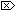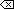The Open Group Base Specifications Issue 6
IEEE Std 1003.1-2001

#### NAME

remquo, remquof, remquol - remainder functions

#### SYNOPSIS

```#include <math.h> double remquo(double x, double y, int *quo); float remquof(float x, float y, int *quo); long double remquol(long double x, long double y, int *quo); ```

#### DESCRIPTION

[CX]The functionality described on this reference page is aligned with the ISO C standard. Any conflict between the requirements described here and the ISO C standard is unintentional. This volume of IEEE Std 1003.1-2001 defers to the ISO C standard.The remquo(), remquof(), and remquol() functions shall compute the same remainder as the remainder(), remainderf(), and remainderl() functions, respectively. In the object pointed to by quo, they store a value whose sign is the sign of x/ y and whose magnitude is congruent modulo 2n to the magnitude of the integral quotient of x/ y, where n is an implementation-defined integer greater than or equal to 3.

An application wishing to check for error situations should set errno to zero and call feclearexcept(FE_ALL_EXCEPT) before calling these functions. On return, if errno is non-zero or fetestexcept(FE_INVALID | FE_DIVBYZERO | FE_OVERFLOW | FE_UNDERFLOW) is non-zero, an error has occurred.

#### RETURN VALUE

These functions shall return x REM y.

[MX]If x or y is NaN, a NaN shall be returned.

If x is ±Inf or y is zero and the other argument is non-NaN, a domain error shall occur, and either a NaN (if supported), or an implementation-defined value shall be returned.#### ERRORS

These functions shall fail if:

Domain Error
[MX]The x argument is ±Inf, or the y argument is ±0 and the other argument is non-NaN.

If the integer expression (math_errhandling & MATH_ERRNO) is non-zero, then errno shall be set to [EDOM]. If the integer expression (math_errhandling & MATH_ERREXCEPT) is non-zero, then the invalid floating-point exception shall be raised.The following sections are informative.

None.

#### APPLICATION USAGE

On error, the expressions (math_errhandling & MATH_ERRNO) and (math_errhandling & MATH_ERREXCEPT) are independent of each other, but at least one of them must be non-zero.

#### RATIONALE

These functions are intended for implementing argument reductions which can exploit a few low-order bits of the quotient. Note that x may be so large in magnitude relative to y that an exact representation of the quotient is not practical.

None.